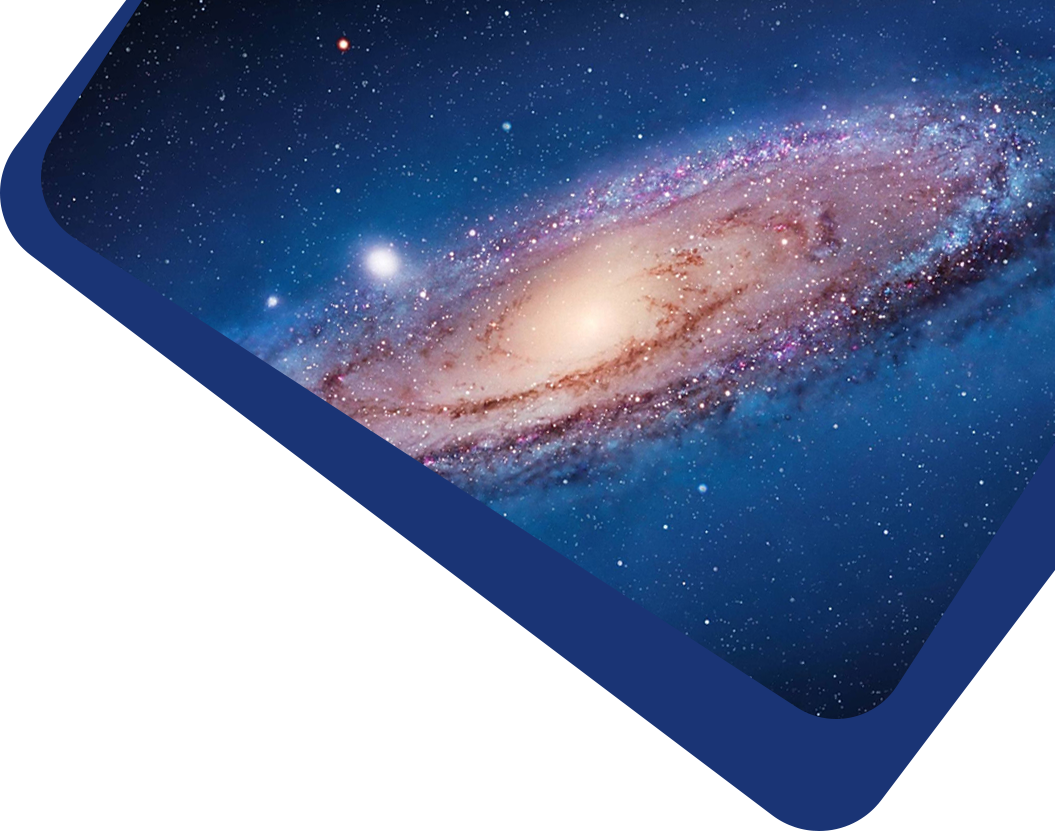Vol 22, No 1

## Alpha/proton Instability in the Presence of Proton and Alpha Temperature Anisotropy and its Application to the Deceleration of Alpha Particles in the Solar Wind

#### Wen-Lu Zhang, Liang Xiang, Qiu-Huan Li, Si-Yi Lang, Hong-Wei Yu

Abstract

Abstract Observations of solar wind plasma find that the drift velocity between alpha beams and protons, $$\nu_{\alpha}$$, is reduced with the heliocentric distance, but the ratio of $$\nu_{\alpha}$$ to $$\nu_A$$ (the local Alfvén velocity) does not vary obviously and has a typical velocity ratio of $$\nu_{\alpha}/\nu_A \le 1$$. The alpha beam instability is believed to be responsible for the deceleration of alpha beams in the solar wind. However, the role of the temperature anisotropy of ions (protons and alpha particles) on the alpha beam instability has not been examined. Based on the kinetic theory of the PDRK solver, this study systemically investigates the alpha beam instability in the presence of proton and alpha temperature anisotropies and considers the effects of these temperature anisotropies on the instability at an arbitrary propagation angle relative to the ambient magnetic field. It is found that the real frequencies, growth rates and threshold conditions of the excited waves sensitively depend on the proton temperature anisotropy $$T_{i\perp}/T_{i\parallel}$$ and the alpha temperature anisotropy $$T_{\alpha \perp}/T_{\alpha\parallel}$$, as well as the parallel electron beta $$\beta_{e\parallel}$$. In particular, for both alpha beam and proton temperature anisotropy driven cases, the parallel magnetosonic/ whistler (PM/W), backward magnetosonic/ whistler (BM/W) and oblique Alfvén/ion cyclotron (OA/IC) waves arise when $$T_{i\perp}/T_{i\parallel}<1$$, and the PM/W, OA/IC, parallel Alfvén/ ion cyclotron (PA/IC) and mirror waves occur when $$T_{i\perp}/T_{i\parallel}>1$$. The presence of anisotropic protons leads to a lower velocity threshold for the OA/IC and BM/W waves at $$T_{i\perp}/T_{i\parallel}<1$$ and for the OA/IC, PA/IC and mirror waves at $$T_{i\perp}/T_{i\parallel}>1$$. For both alpha beam and alpha temperature anisotropy driven cases, the PM/W and OA/IC waves are unstable when $$T_{\alpha \perp}/T_{\alpha\parallel}<1$$, and the PM/W, OA/IC, PA/IC, oblique magnetosonic/ whistler (OM/W) and mirror waves are likely to grow as $$T_{\alpha \perp}/T_{\alpha\parallel}>1$$. The presence of anisotropic alpha particles results in a lower threshold velocity for the PM/W wave at $$T_{\alpha \perp}/T_{\alpha\parallel}<1$$, and for the PA/IC, OM/W and mirror waves at $$T_{\alpha \perp}/T_{\alpha\parallel}>1$$. On account of the influences of the anisotropic proton and alpha, these alpha beam instabilities can effectively constrain the alpha-beam drift velocity to less than or equal to the local Alfvén velocity. These results may shed light on the evolution and deceleration mechanism of alpha particles in the solar wind.

Keywords

Keywords (Sun:) solar wind – Sun: heliosphere – waves

Full Text
Refbacks

• There are currently no refbacks.